Picture by Christian Hume, Unsplash.

Line charts, line graphs, or line plots are among the most widely used data visualisations. They’re ideal for time series data in which you’re plot a metric on the y axis over a time period on the X axis and are quick and easy to produce and intuitive to read.

Seaborn and Matplotlib make it easy to take your Pandas data and plot it on line plots that you can use during Exploratory Data Analysis, in your reports and presentations, or in online applications. Here’s how it’s done.

For this project we’re using Pandas, Seaborn, and Matplotlib for displaying the data. I’m also using GAPandas to fetch web analytics data from my Google Analytics account using the API, but you can use any data source you like. Open up a Jupyter notebook and load the packages.

``````import pandas as pd
import seaborn as sns
import matplotlib.pyplot as plt
from gapandas import connect, query
``````

### Set up retina mode

To make the Seaborn charts look better on larger screens and in Jupyter notebooks, I’ve passed in the `retina` option to Seaborn and used the `set_context()` function to tell Seaborn it’s displaying charts in a Jupyter notebook.

``````%config InlineBackend.figure_format = 'retina'
sns.set_context('notebook')
``````

### Setting the lineplot size

To control the sizes of the lineplots throughout the notebook I’ve used `set()` to define the figure size as 15 inches by 6 inches. You can override this on a per chart basis, should you need to change it, but this will save code repetition below.

``````sns.set(rc={'figure.figsize':(15, 6)})
``````

### Fetch the data

Next, we’ll use GAPandas to fetch data from my Google Analytics account. Using GAPandas is covered in this article so you can follow that to get set up if you want to use your own data as the source. The Google Analytics API query used selects sessions by day over the past few years.

``````service = connect.get_service('personal_client_secrets.json')
``````
``````payload = {
'start_date': '2017-01-01',
'end_date': '2020-10-31',
'metrics': 'ga:sessions',
'dimensions': 'ga:date'
}

df_daily['date'] = pd.to_datetime(df_daily['date'])
df_daily['sessions'] = df_daily['sessions'].astype(int)
``````
date sessions
0 2017-01-01 130
1 2017-01-02 137
2 2017-01-03 126
3 2017-01-04 143
4 2017-01-05 153

### Line plot

To create a simple line plot using Seaborn we can simply call the `lineplot()` function and pass in the name of the `x` (horizontal) axis column, the name of the `y` (vertical) axis column, and the name of the dataframe. This creates a single line plot.

``````line = sns.lineplot(x='date',
y='sessions',
data=df_daily)
``````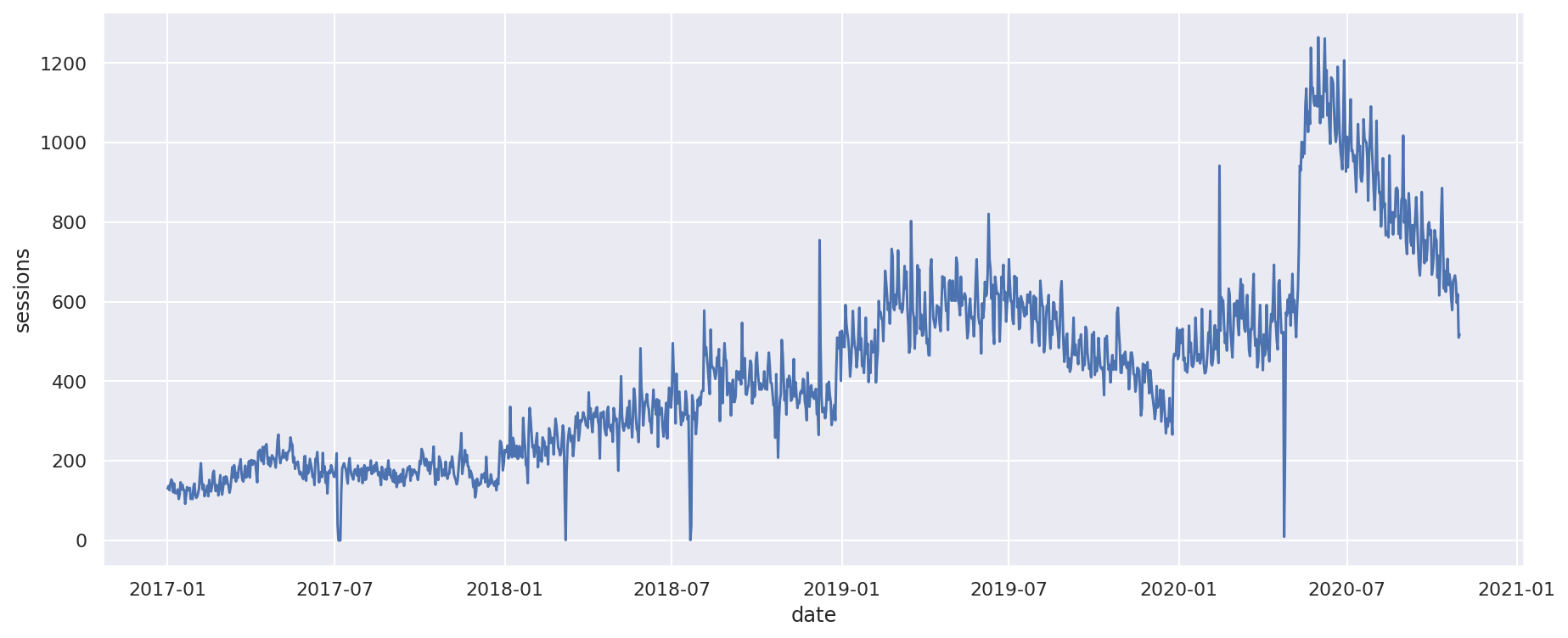#### Rotating axis labels

By default, the axis labels (or ticks) are horizontal, so they can overlap on smaller graphs. To rotate the axis labels we can use a bit of Matplotlib code to rotate them 90 degrees so they sit vertically.

``````line = sns.lineplot(x='date',
y='sessions',
data=df_daily)
plt.xticks(rotation=90)
plt.tight_layout()
``````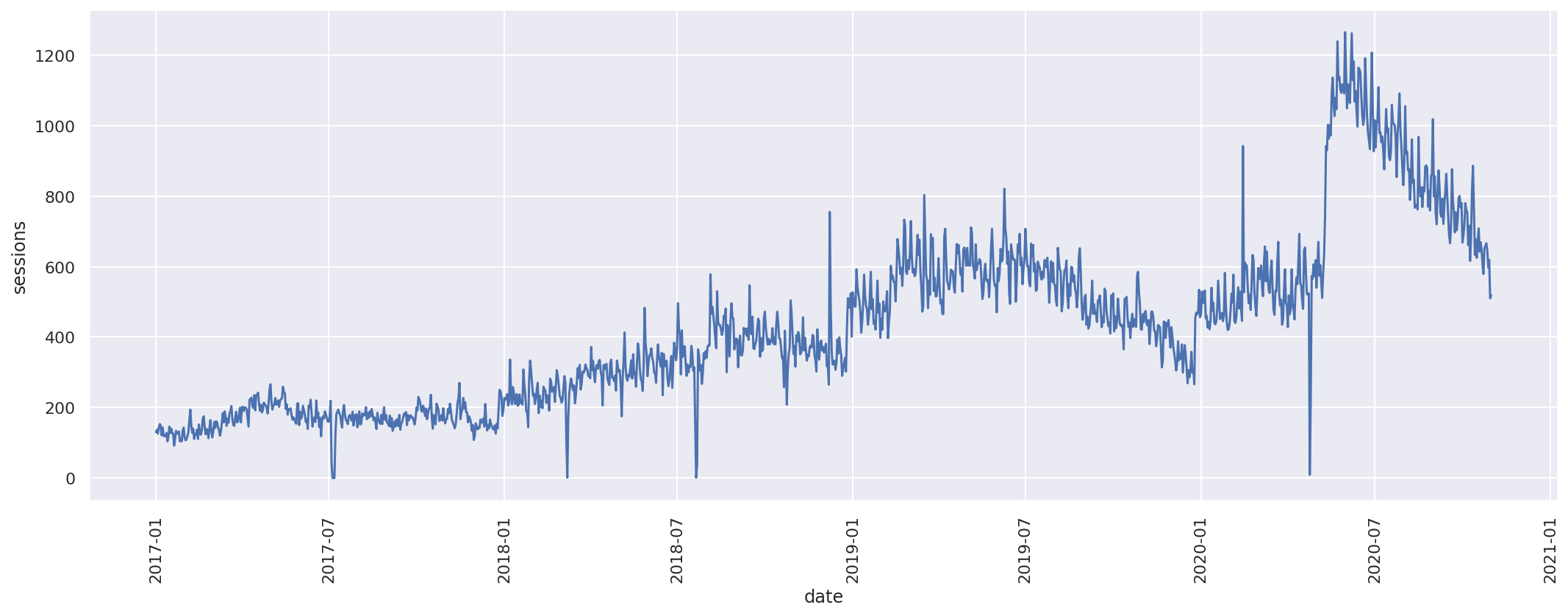### Multi-line plot

The line plot created above only has one line. However, it’s very common to need to plot multiple lines, so let’s create another data set including time series data for each of a range of channels. This time, we’ll group the data on the `ga:yearMonth` dimension to obtain a monthly data set and we will add the `ga:medium` dimension and use a regular expression filter to select only the direct, referral, social or email data.

``````payload = {
'start_date': '2017-01-01',
'end_date': '2020-10-31',
'metrics': 'ga:sessions',
'dimensions': 'ga:yearMonth, ga:medium',
'filters': 'ga:medium=~direct|referral|social|email'
}

df_monthly['sessions'] = df_monthly['sessions'].astype(int)
``````

Creating a multi line plot in Seaborn is just as easy. The `x` axis is set to the `yearMonth`, the `y` is set to the `sessions`, and the `medium` is set as the `hue` value. This will plot the data for each `medium` on its own line and add a legend to show which coloured line is which.

``````multi = sns.lineplot(x='yearMonth',
y='sessions',
hue='medium',
data=df_monthly)
plt.xticks(rotation=90)
plt.tight_layout()
``````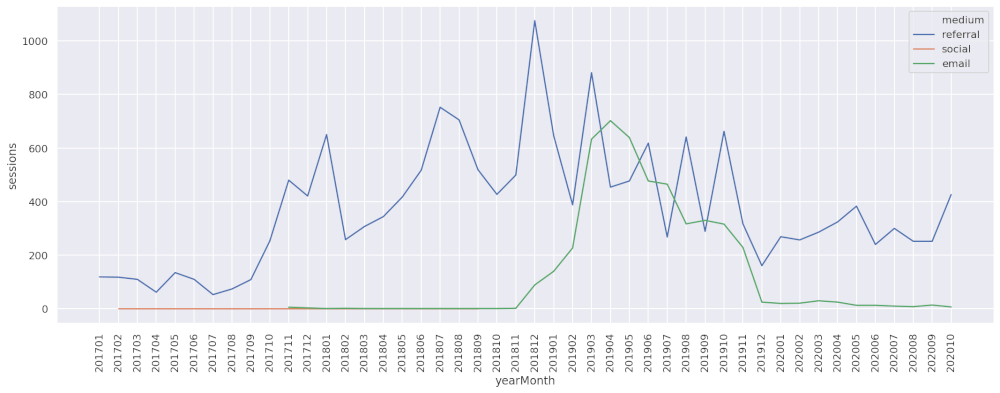#### Setting the plot size

As I mentioned above, we’ve set a default plot size using `figsize()` for the whole notebook, but you can override this using `fig, ax = plt.subplots(figsize=(15,5))` where the first value is the width in inches and the second is the height in inches.

``````fig, ax = plt.subplots(figsize=(15,5))

multi = sns.lineplot(x='yearMonth',
y='sessions',
hue='medium',
data=df_monthly)
plt.xticks(rotation=90)
plt.tight_layout()
``````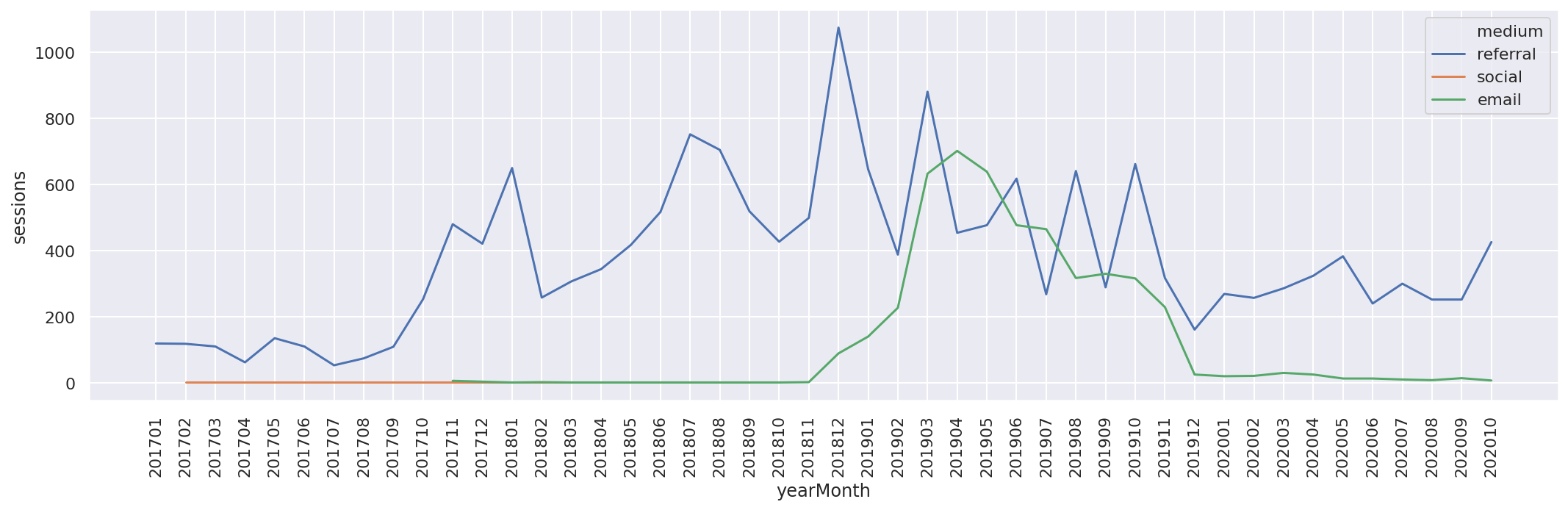To add a title and set the font to size 15 and font weight to bold, we can use the Matplotlib `title()` function. This puts a nice clear title above the plot.

``````plt.title('Monthly sessions', fontsize=15, fontweight="bold")
multi = sns.lineplot(x='yearMonth',
y='sessions',
hue='medium',
data=df_monthly)
plt.xticks(rotation=90)
plt.tight_layout()
``````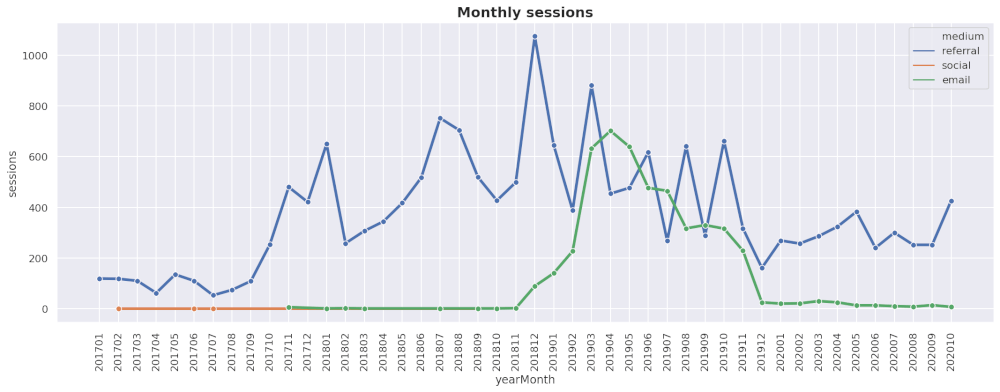You can add axis labels and set their font weights using a similar approach. To control the axis label text and colour you need to use `set_xlabel()` and `set_ylabel()` which are accessible via `ax`.

``````fig, ax = plt.subplots(figsize=(15,5))

multi = sns.lineplot(x='yearMonth',
y='sessions',
hue='medium',
data=df_monthly)
plt.xticks(rotation=90)
plt.tight_layout()

ax.set_xlabel('Month', fontweight='bold')
ax.set_ylabel('Sessions', fontweight='bold')
``````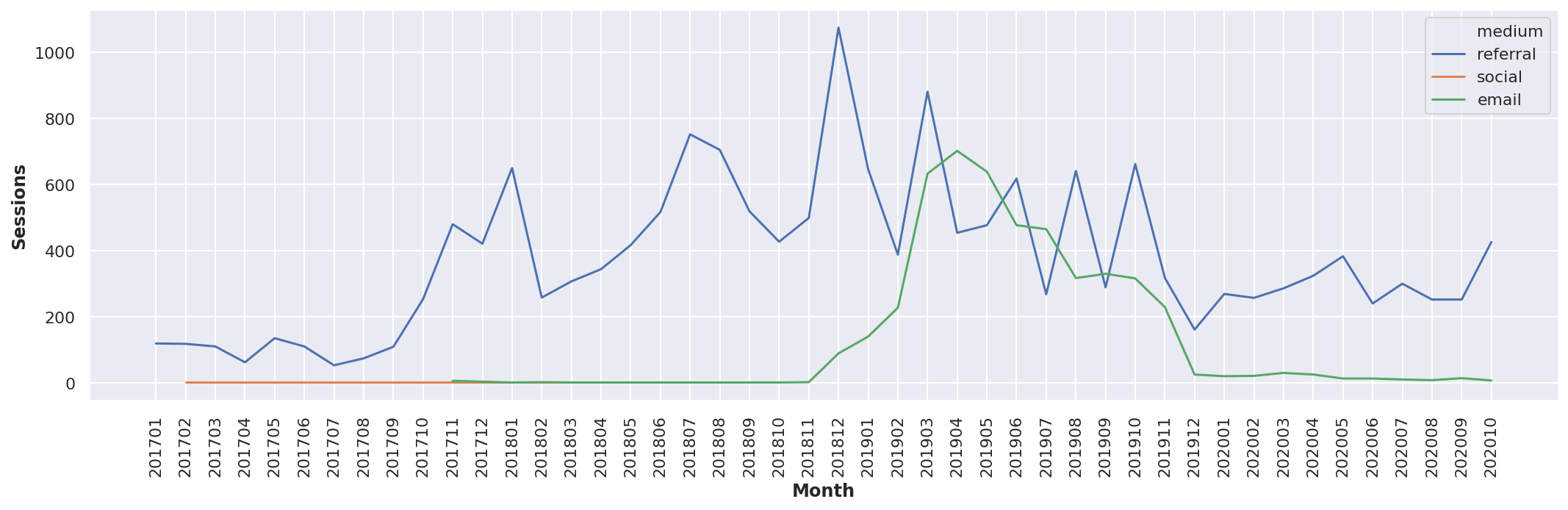Sometimes it’s easier to read line plots when there are dots to mark where each point lines up with one of the dates. These are really easy to add. Simply put `marker='o'` in the `lineplot()` function arguments and it will add little marker dots.

``````multi = sns.lineplot(x='yearMonth',
y='sessions',
hue='medium',
marker='o',
data=df_monthly)
plt.xticks(rotation=90)
plt.tight_layout()
``````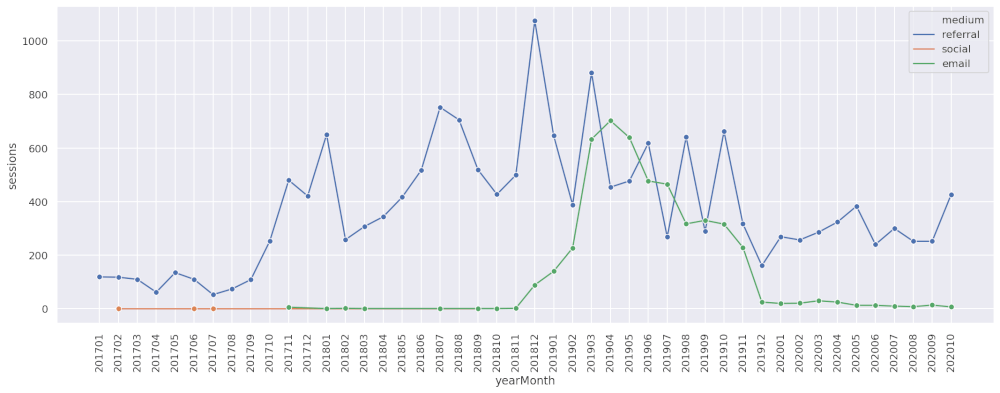#### Setting line thickness

Making the lines on your plot thicker can also make them easier to read, especially if you’re using them in a PowerPoint presentation. Line thickness is controlled via the `linewidth` argument, which takes an integer value for the line width in pixels.

``````multi = sns.lineplot(x='yearMonth',
y='sessions',
hue='medium',
marker='o',
linewidth=3,
data=df_monthly)
plt.xticks(rotation=90)
plt.tight_layout()
``````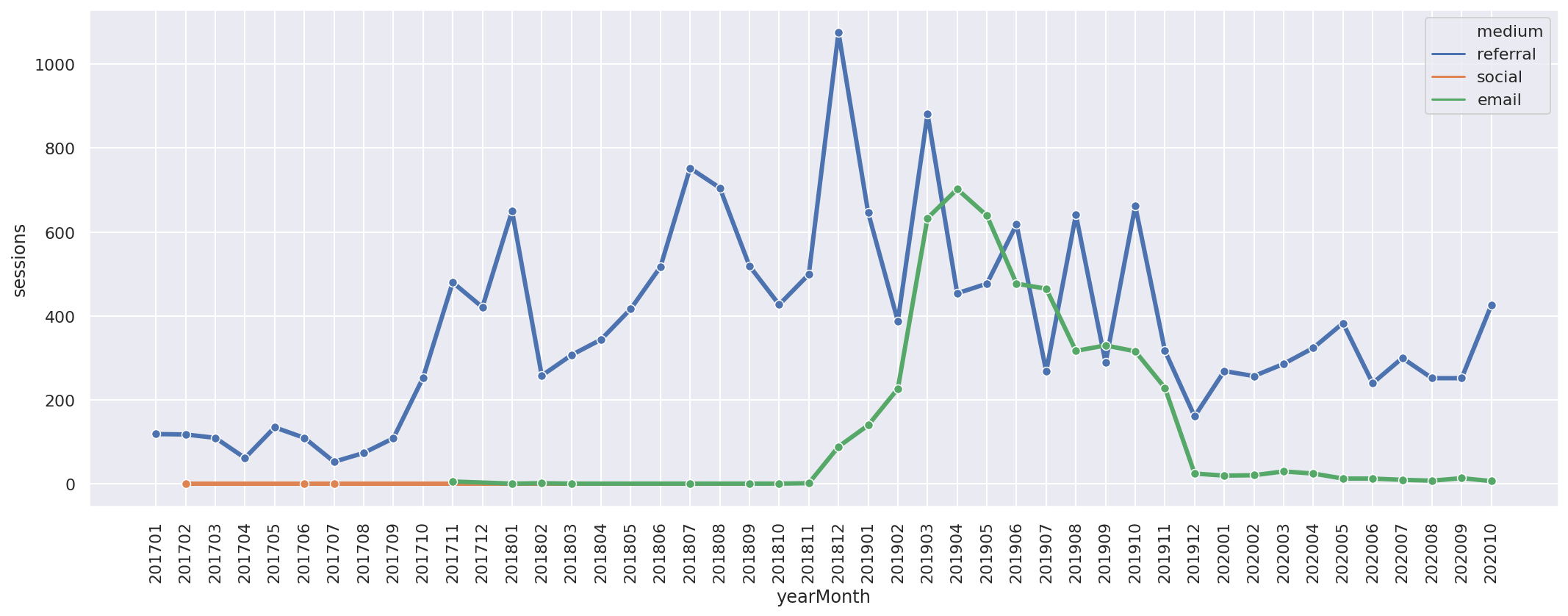### Lineplot theming

Seaborn comes with quite a good selection of themes to allow you to make your charts look more stylish than the default setting. To apply one of the standard Seaborn style themes you simply call `set_style()` and enter the name of the theme as the argument. Here’s a selection of common Seaborn themes.

#### Whitegrid theme

``````sns.set_style('whitegrid')
multi = sns.lineplot(x='yearMonth',
y='sessions',
hue='medium',
marker='o',
linewidth=3,
data=df_monthly)
plt.xticks(rotation=90)
plt.tight_layout()
``````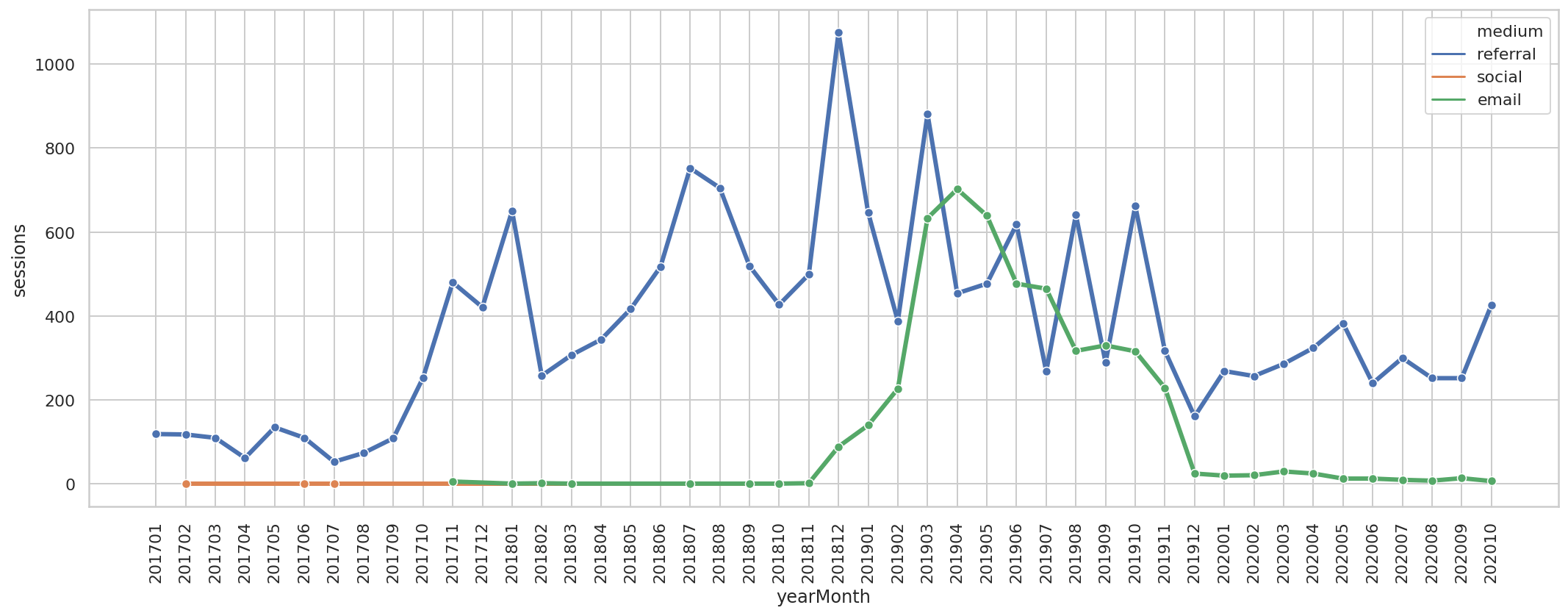#### Dark theme

``````sns.set_style('dark')
multi = sns.lineplot(x='yearMonth',
y='sessions',
hue='medium',
marker='o',
linewidth=3,
data=df_monthly)
plt.xticks(rotation=90)
plt.tight_layout()
``````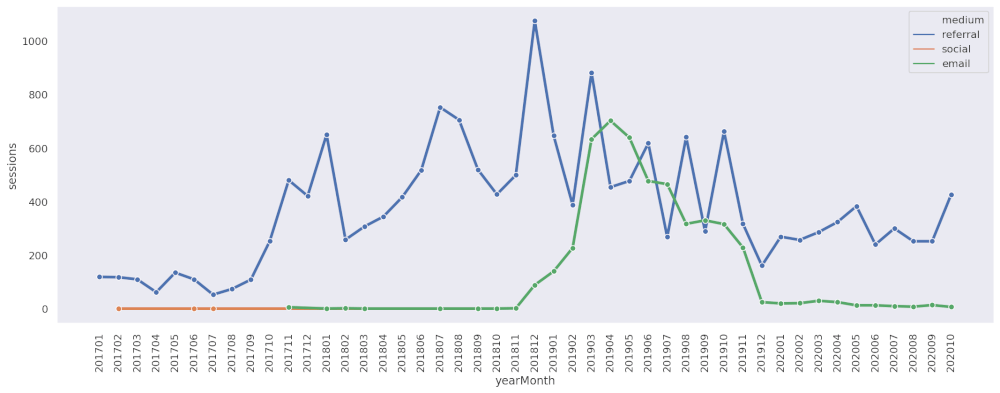#### White theme

``````sns.set_style('white')
multi = sns.lineplot(x='yearMonth',
y='sessions',
hue='medium',
marker='o',
linewidth=3,
data=df_monthly)
plt.xticks(rotation=90)
plt.tight_layout()
``````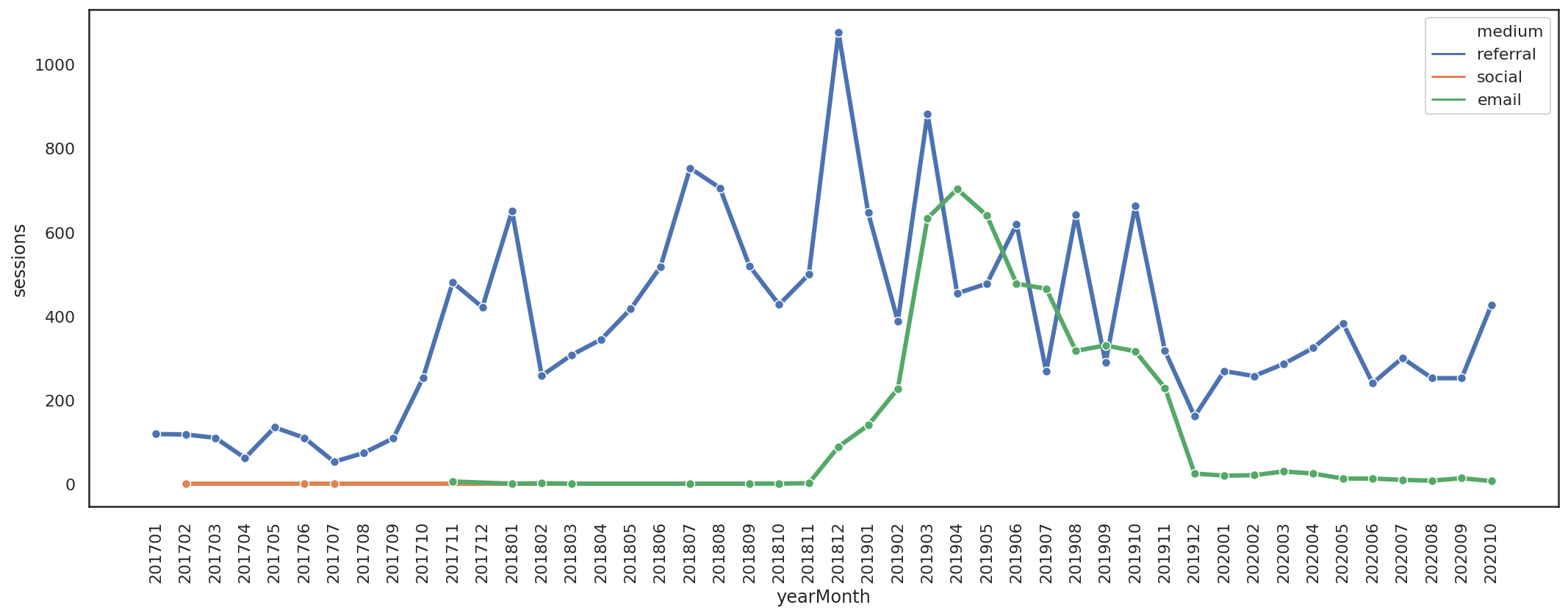#### Ticks theme

``````sns.set_style('ticks')
multi = sns.lineplot(x='yearMonth',
y='sessions',
hue='medium',
marker='o',
linewidth=3,
data=df_monthly)
plt.xticks(rotation=90)
plt.tight_layout()
``````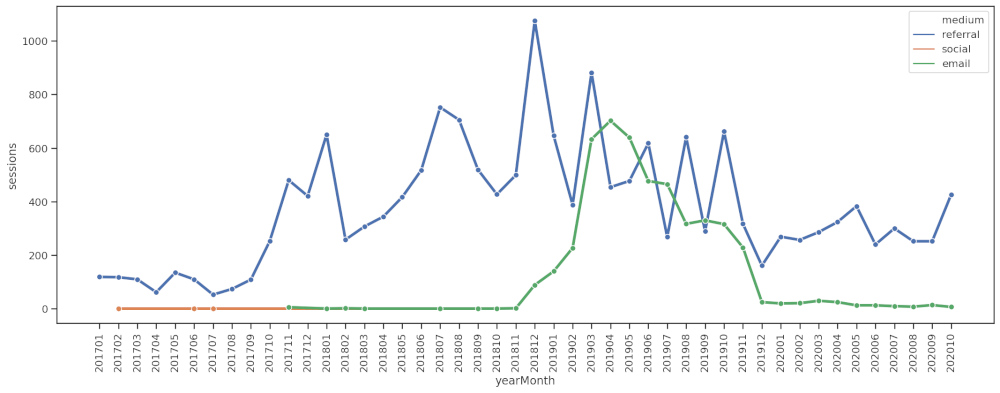### Lineplot colour palettes

As well as themes, Seaborn also lets you control the colour palette, which dictates the colours used for adding the lines. You can change the line colour palette by adding the `palette` argument to `lineplot()` and entering one of the standard palette names. Here’s a selection of them.

#### Deep palette

``````sns.set_style('white')
multi = sns.lineplot(x='yearMonth',
y='sessions',
hue='medium',
marker='o',
linewidth=3,
data=df_monthly,
palette='deep')
plt.xticks(rotation=90)
plt.tight_layout()
``````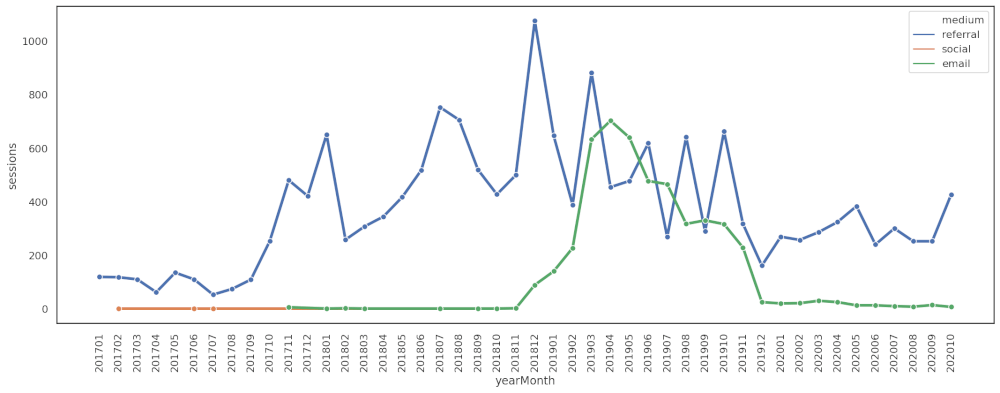#### Muted palette

``````sns.set_style('white')
multi = sns.lineplot(x='yearMonth',
y='sessions',
hue='medium',
marker='o',
linewidth=3,
data=df_monthly,
palette='muted')
plt.xticks(rotation=90)
plt.tight_layout()
``````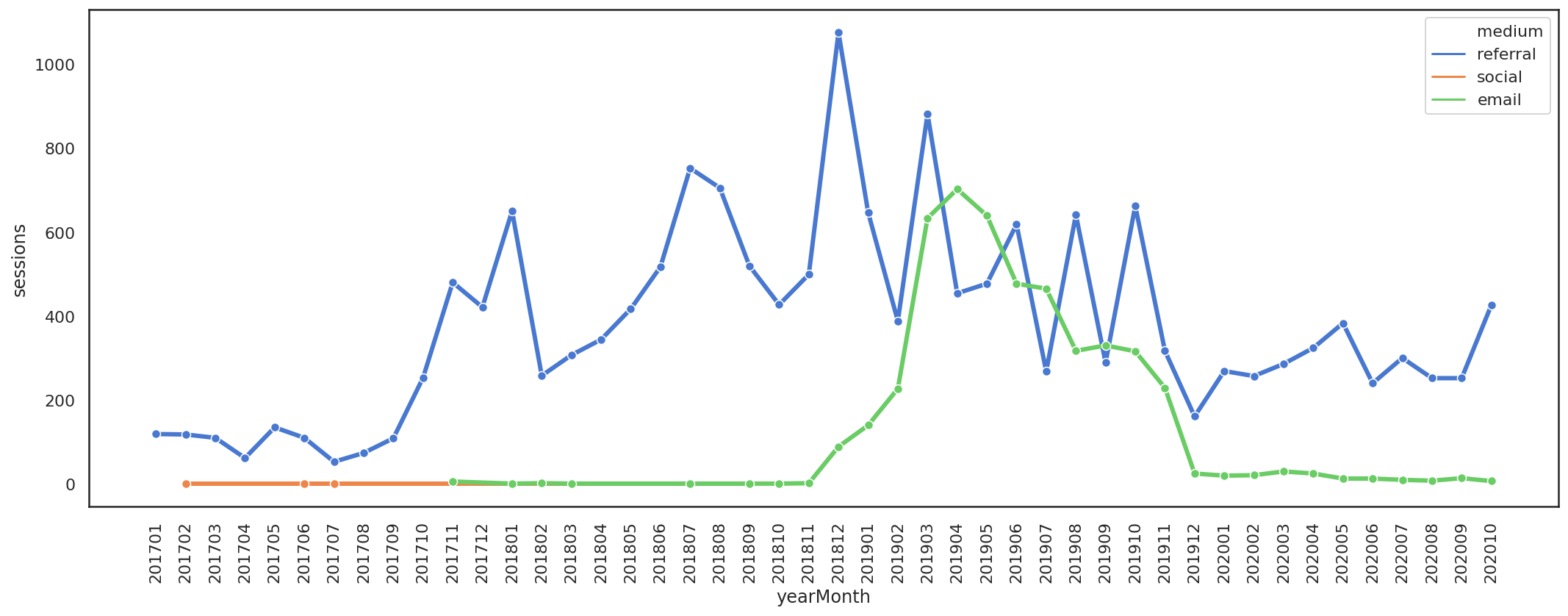#### Pastel palette

``````sns.set_style('white')
multi = sns.lineplot(x='yearMonth',
y='sessions',
hue='medium',
marker='o',
linewidth=3,
data=df_monthly,
palette='pastel')
plt.xticks(rotation=90)
plt.tight_layout()
``````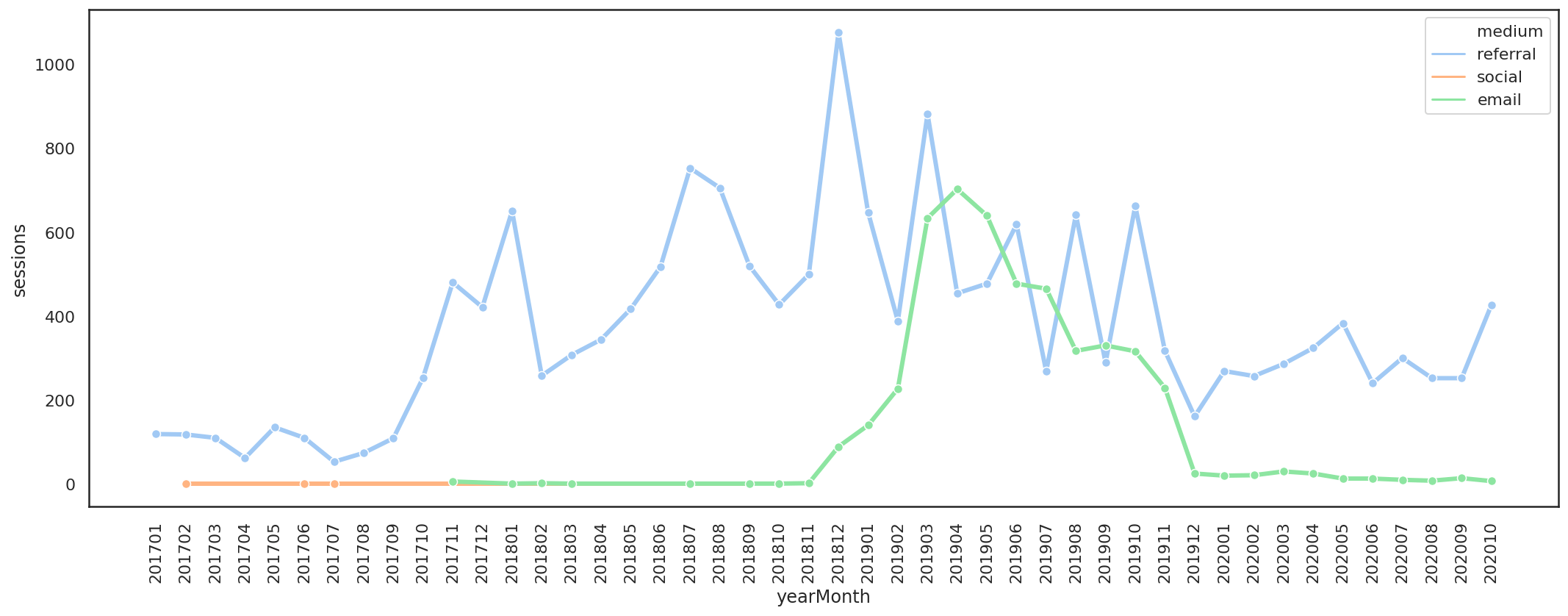#### Bright palette

``````sns.set_style('white')
multi = sns.lineplot(x='yearMonth',
y='sessions',
hue='medium',
marker='o',
linewidth=3,
data=df_monthly,
palette='bright')
plt.xticks(rotation=90)
plt.tight_layout()
``````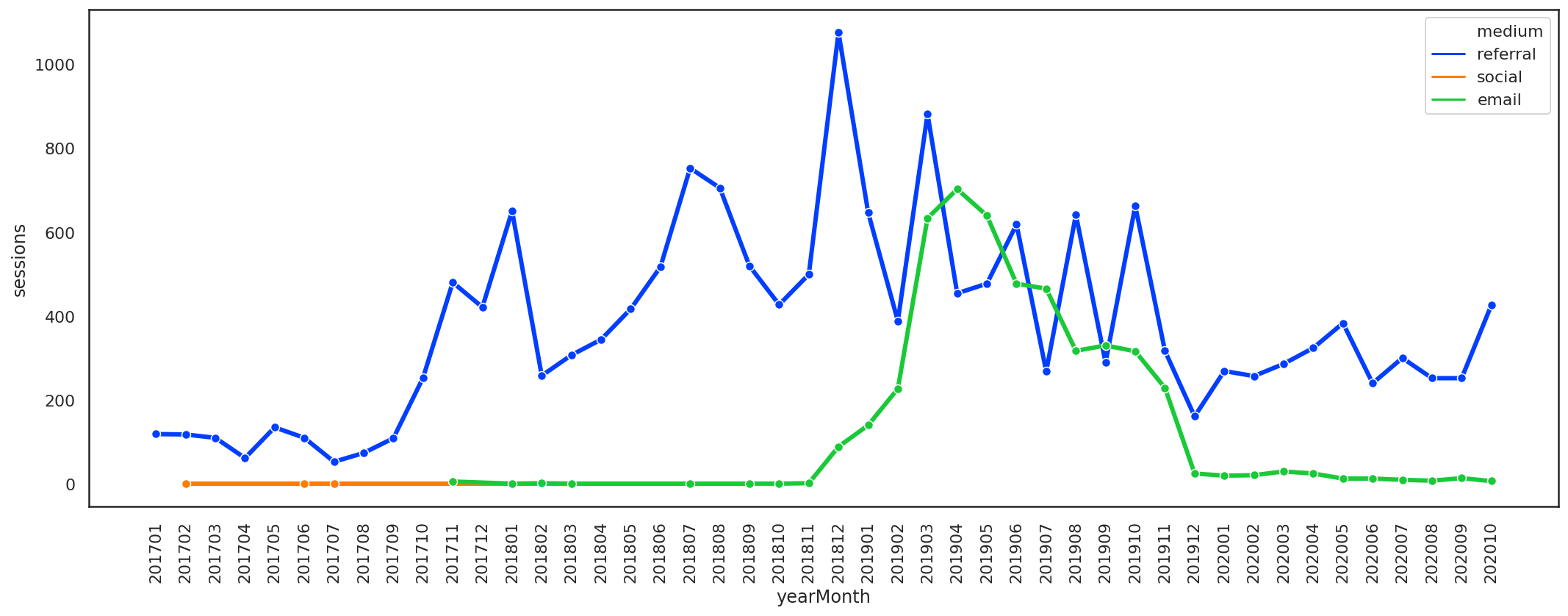#### Colorblind palette

``````sns.set_style('white')
multi = sns.lineplot(x='yearMonth',
y='sessions',
hue='medium',
marker='o',
linewidth=3,
data=df_monthly,
palette='colorblind')
plt.xticks(rotation=90)
plt.tight_layout()
``````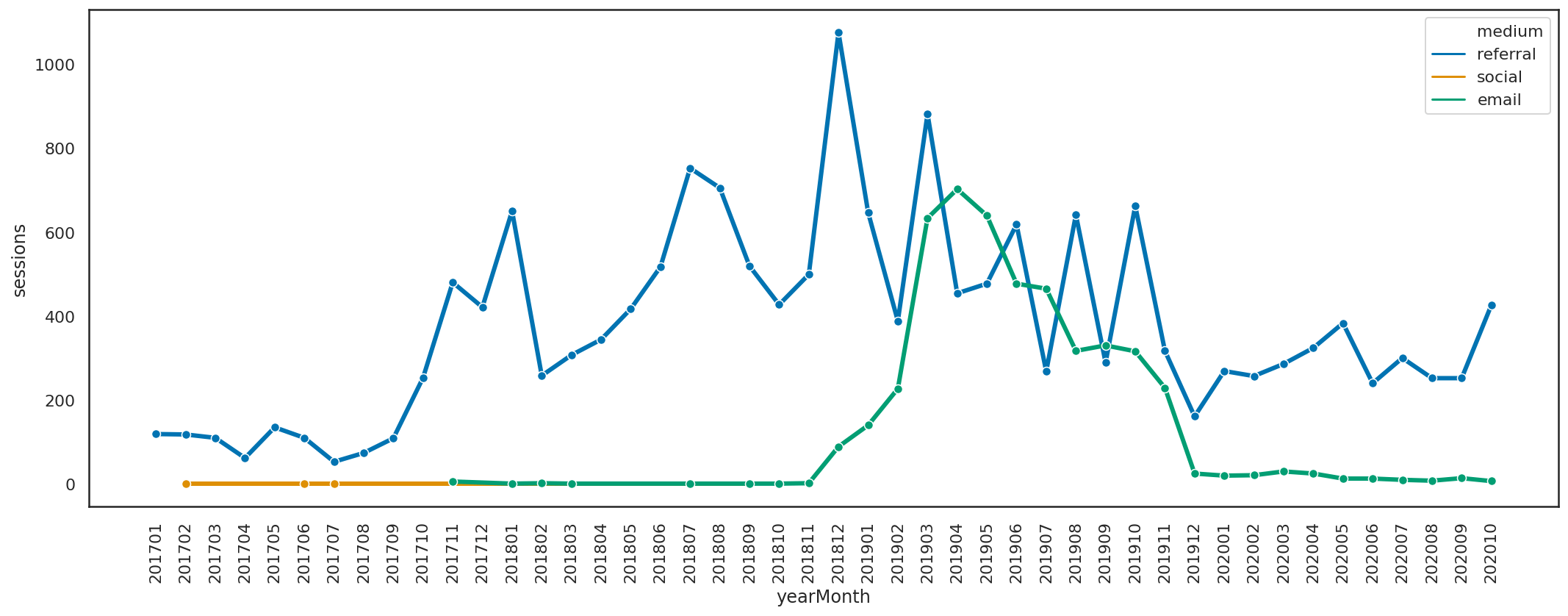#### Husl (HSLuv) palette

``````sns.set_style('white')
multi = sns.lineplot(x='yearMonth',
y='sessions',
hue='medium',
marker='o',
linewidth=3,
data=df_monthly,
palette='husl')
plt.xticks(rotation=90)
plt.tight_layout()
``````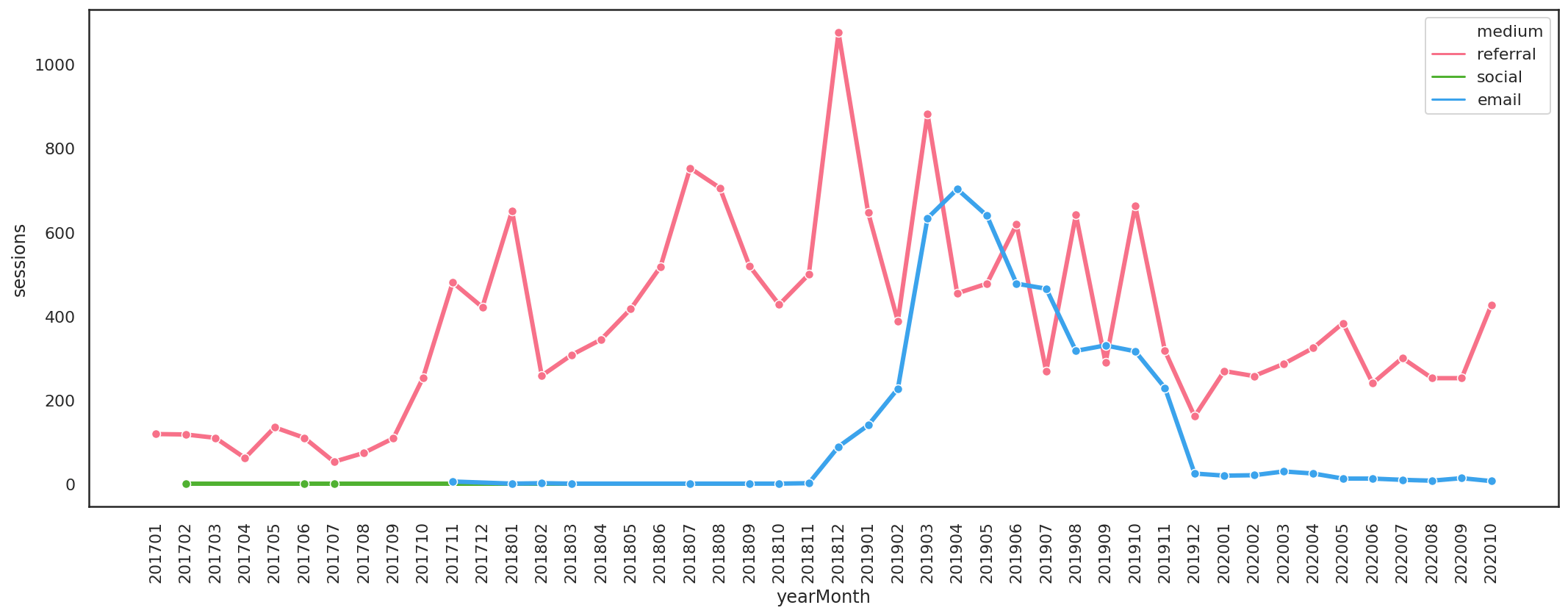### Other tricks

#### Removing borders or “despining”

There are other little tricks you can apply to Seaborn line charts to control their appearance. De-spining removes the border or line around the edge of the line chart. These are controlled via the `despine()` function with an argument telling Seaborn which spine to remove.

``````sns.set_style('white')
multi = sns.lineplot(x='yearMonth',
y='sessions',
hue='medium',
marker='o',
linewidth=3,
data=df_monthly,
palette='husl')
plt.xticks(rotation=90)
plt.tight_layout()

sns.despine(top=True, left=True, bottom=True)
``````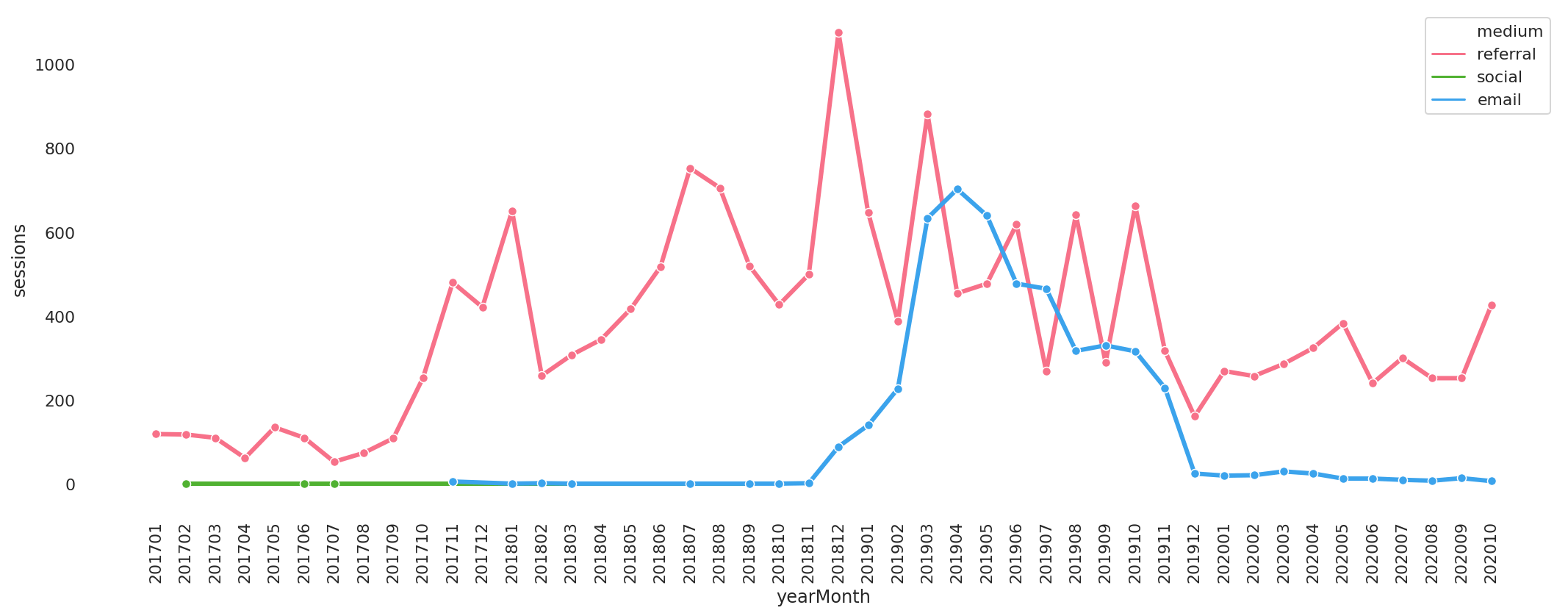#### Setting custom colours

As well as the standard Seaborn colour palettes, you can also define your own. To do this you create a list of colours to use, assign these using `colour_palette()` and then add that to `set_palette()`.

``````colours = ['#32B5C9','#7B70BA','#A23565']
custom_palette = sns.set_palette(sns.color_palette(colours))

multi = sns.lineplot(x='yearMonth',
y='sessions',
hue='medium',
marker='o',
linewidth=5,
data=df_monthly,
palette=custom_palette)

ax.set_xlabel('Month')
ax.set_ylabel('Sessions')

plt.title('Monthly sessions', fontsize=15, fontweight='bold')
plt.xticks(rotation=90)
plt.tight_layout()

sns.despine(top=True, left=True, bottom=True)
``````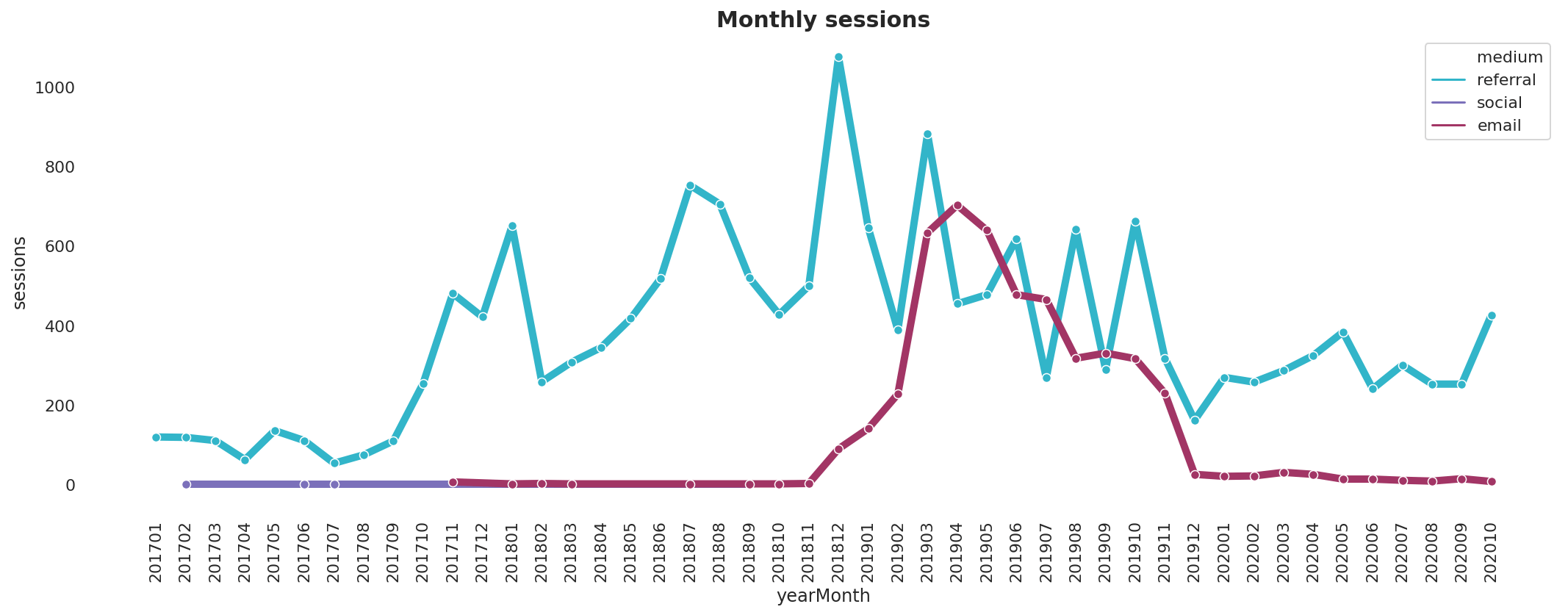Matt Clarke, Sunday, March 07, 2021

Matt Clarke Matt is an Ecommerce and Marketing Director who uses data science to help in his work. Matt has a Master's degree in Internet Retailing (plus two other Master's degrees in different fields) and specialises in the technical side of ecommerce and marketing.